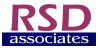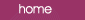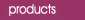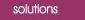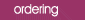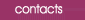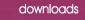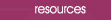Effect Plots Two Effect Plots which are described by Wheeler & Lyday are generated by Mesa: (i) the Main Effect Plot for Inconsistency and (ii) the Main Effect Plot for Bias. The upper plot  (obtained using the AIAG data) is basically an Analysis of Mean Ranges (ANOMR) plot, a method which has also been described by Ullman.  The decision limits shown are the 5% limits shown on the Calculations sheet for operator ranges (0.021965, 0.056963).  There are no points outside the limits and this indicates that there are no significant differences in the variation of each operators measurements.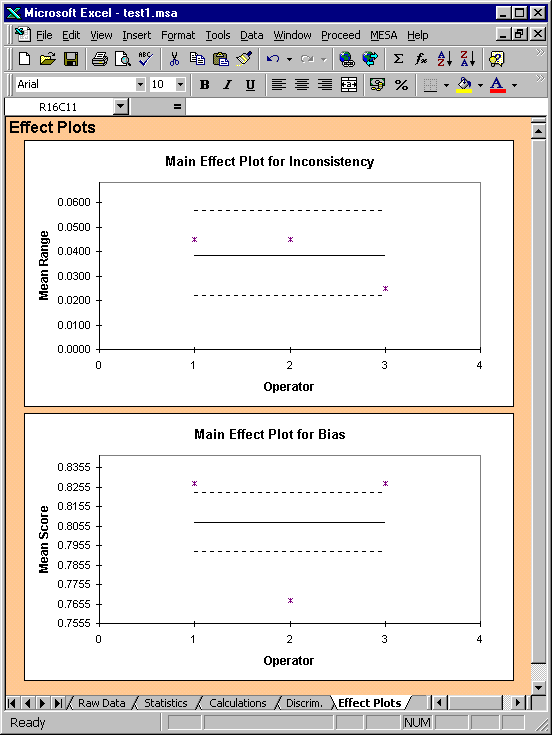The lower chart shows the Main Effect Plot for Bias for the AIAG data. This is basically an Analysis of Means (ANOM) plot.  In this example the plot indicates that there is a statistically significant difference between the means of the different operators. The Effect Plots are one of the chart types which are fundamental to the Wheeler & Lyday approach.  The plots above show how easy it is to pick out differences between operators (or Test Stations, or Laboratories etc).  Therefore these plots help us to identify assignable causes of variation.